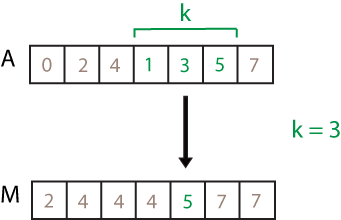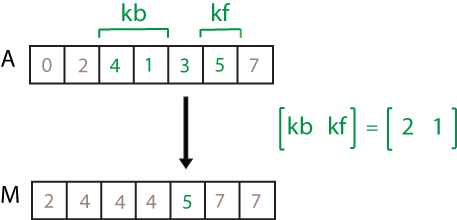# movmax

Moving maximum

## Syntax

``M = movmax(A,k)``
``M = movmax(A,[kb kf])``
``M = movmax(___,dim)``
``M = movmax(___,nanflag)``
``M = movmax(___,Name,Value)``

## Description

example

````M = movmax(A,k)` returns an array of local `k`-point maximum values, where each maximum is calculated over a sliding window of length `k` across neighboring elements of `A`. When `k` is odd, the window is centered about the element in the current position. When `k` is even, the window is centered about the current and previous elements. The window size is automatically truncated at the endpoints when there are not enough elements to fill the window. When the window is truncated, the maximum is taken over only the elements that fill the window. `M` is the same size as `A`.If `A` is a vector, then `movmax` operates along the length of the vector `A`.If `A` is a multidimensional array, then `movmax` operates along the first dimension of `A` whose size does not equal 1.```

example

````M = movmax(A,[kb kf])` computes the maximum with a window of length `kb+kf+1` that includes the element in the current position, `kb` elements backward, and `kf` elements forward.```

example

````M = movmax(___,dim)` specifies the dimension of `A` to operate along for any of the previous syntaxes. For example, if `A` is a matrix, then `movmax(A,k,2)` operates along the columns of `A`, computing the `k`-element sliding maximum for each row.```

example

````M = movmax(___,nanflag)` specifies whether to omit or include `NaN` values in `A`. For example, `movmax(A,k,"includenan")` includes all `NaN` values when computing each maximum. By default, `movmax` omits `NaN` values.```

example

````M = movmax(___,Name,Value)` specifies additional parameters for the moving maximum using one or more name-value arguments. For example, if `x` is a vector of time values, then `movmax(A,k,"SamplePoints",x)` computes the moving maximum relative to the times in `x`.```

## Examples

collapse all

Compute the three-point centered moving maximum of a row vector. When there are fewer than three elements in the window at the endpoints, take the maximum over the elements that are available.

```A = [4 8 6 -1 -2 -3 -1 3 4 5]; M = movmax(A,3)```
```M = 1×10 8 8 8 6 -1 -1 3 4 5 5 ```

Compute the three-point trailing moving maximum of a row vector. When there are fewer than three elements in the window at the endpoints, `movmax` takes the maximum over the number of elements that are available.

```A = [4 8 6 -1 -2 -3 -1 3 4 5]; M = movmax(A,[2 0])```
```M = 1×10 4 8 8 8 6 -1 -1 3 4 5 ```

Compute the three-point centered moving maximum for each row of a matrix. The window starts on the first row, slides horizontally to the end of the row, then moves to the second row, and so on. The dimension argument is two, which slides the window across the columns of `A`.

`A = [4 8 6; -1 -2 -3; -1 3 4]`
```A = 3×3 4 8 6 -1 -2 -3 -1 3 4 ```
`M = movmax(A,3,2)`
```M = 3×3 8 8 8 -1 -1 -2 3 4 4 ```

Create a row vector containing `NaN` values.

`A = [4 8 NaN -1 -2 -3 NaN 3 4 5];`

Compute the three-point centered moving maximum values of the vector, including `NaN` values. For windows that contain any `NaN` value, the corresponding maximum value is `NaN`.

`M = movmax(A,3,"includenan")`
```M = 1×10 8 NaN NaN NaN -1 NaN NaN NaN 5 5 ```

Compute a 3-hour centered moving maximum of the data in `A` according to the time vector `t`.

```A = [4 8 6 -1 -2 -3]; k = hours(3); t = datetime(2016,1,1,0,0,0) + hours(0:5)```
```t = 1x6 datetime 01-Jan-2016 00:00:00 01-Jan-2016 01:00:00 01-Jan-2016 02:00:00 01-Jan-2016 03:00:00 01-Jan-2016 04:00:00 01-Jan-2016 05:00:00 ```
`M = movmax(A,k,"SamplePoints",t)`
```M = 1×6 8 8 8 6 -1 -2 ```

Compute the three-point centered moving maximum of a row vector, but discard any calculation that uses fewer than three points from the output. In other words, return only the maximums computed from a full three-element window, discarding endpoint calculations.

```A = [4 8 6 -1 -2 -3 -1 3 4 5]; M = movmax(A,3,"Endpoints","discard")```
```M = 1×8 8 8 6 -1 -1 3 4 5 ```

## Input Arguments

collapse all

Input array, specified as a vector, matrix, or multidimensional array.

Data Types: `single` | `double` | `int8` | `int16` | `int32` | `int64` | `uint8` | `uint16` | `uint32` | `uint64` | `logical`

Window length, specified as a numeric or duration scalar. When `k` is a positive integer scalar, the centered maximum includes the element in the current position plus surrounding neighbors.

For example, `movmax(A,3)` computes an array of local three-point maximum values.Directional window length, specified as a numeric or duration row vector containing two elements. When `kb` and `kf` are positive integer scalars, the calculation is over `kb+kf+1` elements. The calculation includes the element in the current position, `kb` elements before the current position, and `kf` elements after the current position.

For example, `movmax(A,[2 1])` computes an array of local four-point maximum values.Dimension to operate along, specified as a positive integer scalar. If you do not specify the dimension, then the default is the first array dimension of size greater than 1.

Dimension `dim` indicates the dimension that `movmax` operates along, that is, the direction in which the specified window slides.

Consider an `m`-by-`n` input matrix, `A`:

• `movmax(A,k,1)` computes the `k`-element sliding maximum for each column of `A` and returns an `m`-by-`n` matrix.• `movmax(A,k,2)` computes the `k`-element sliding maximum for each row of `A` and returns an `m`-by-`n` matrix.Missing value condition, specified as one of these values:

• `"omitmissing"` or `"omitnan"` — Ignore `NaN` values in `A`, and compute each maximum over fewer points. If all elements in a window are `NaN`, then the corresponding element in `M` is `NaN`. `"omitmissing"` and `"omitnan"` have the same behavior.

• `"includemissing"` or `"includenan"` — Include `NaN` values in `A` when computing each maximum. If any element in the window is `NaN`, then the corresponding element in `M` is `NaN`. `"includemissing"` and `"includenan"` have the same behavior.

### Name-Value Arguments

Specify optional pairs of arguments as `Name1=Value1,...,NameN=ValueN`, where `Name` is the argument name and `Value` is the corresponding value. Name-value arguments must appear after other arguments, but the order of the pairs does not matter.

Before R2021a, use commas to separate each name and value, and enclose `Name` in quotes.

Example: `M = movmax(A,k,"Endpoints","fill")`

Method to treat leading and trailing windows, specified as one of these options:

ValueDescription
`"shrink"`Shrink the window size near the endpoints of the input to include only existing elements.
`"discard"`Do not output any maximum values when the window does not completely overlap with existing elements.
`"fill"`Substitute nonexisting elements with `-Inf`.
numeric or logical scalarSubstitute nonexisting elements with a specified numeric or logical value.

Sample points for computing maximums, specified as a vector. The sample points represent the location of the data in `A`. Sample points do not need to be uniformly sampled. By default, the sample points vector is `[1 2 3 ... ]`.

Moving windows are defined relative to the sample points, which must be sorted and contain unique elements. For example, if `t` is a vector of times corresponding to the input data, then `movmax(rand(1,10),3,"SamplePoints",t)` has a window that represents the time interval between `t(i)-1.5` and `t(i)+1.5`.

When the sample points vector has data type `datetime` or `duration`, then the moving window length must have type `duration`.

If the sample points are nonuniformly spaced and `Endpoints` is specified, then its value must be `"shrink"`.

## Version History

Introduced in R2016a

expand all